﻿ Application of Polynomial Regression Models in Prediction of Residual Stresses of a Transversal Beam

### Application of Polynomial Regression Models in Prediction of Residual Stresses of a Transversal Beam

František Trebuňa, Eva Ostertagová, Peter Frankovský, Oskar Ostertag

American Journal of Mechanical Engineering

## Application of Polynomial Regression Models in Prediction of Residual Stresses of a Transversal Beam

František Trebuňa1, Eva Ostertagová2, Peter Frankovský3,, Oskar Ostertag1

1Department of Applied Mechanics and Mechanical Engineering, Faculty of Mechanical Engineering, Technical University of Košice, Letná 9, 042 00 Košice, Slovak Republic

2Department of Mathematics and Theoretical Informatics, Faculty of Electrical Engineering and Informatics, Technical University of Košice, Nemcovej 32, 042 00 Košice, Slovak Republic

3Department of Mechatronics, Faculty of Mechanical Engineering, Technical University of Košice, Letná 9, 042 00 Košice, Slovak Republic

### Abstract

Residual stresses significantly affect the behavior of a structure or its individual components. Residual stresses can be determined experimentally. It should be noted that careful procedure and preparation of the experiment are necessary in order to assess the effects on a specific component. The transversal beam of a casting structure underwent a series of measurements as a result of frequent operational faults due to cracks which used to occur in the vicinity of welds and other areas with high concentration of stresses. The measurement was done with a mechanical method of drilling with the use of a tensiometric rosette. Since the hole was drilled into the material to a specific depth, it was necessary to predict stresses in different depth levels of the hole. The prediction of local deformations was based on methods of mathematical statistics supported by MATLAB.

• František Trebuňa, Eva Ostertagová, Peter Frankovský, Oskar Ostertag. Application of Polynomial Regression Models in Prediction of Residual Stresses of a Transversal Beam. American Journal of Mechanical Engineering. Vol. 4, No. 7, 2016, pp 247-251. http://pubs.sciepub.com/ajme/4/7/3
• Trebuňa, František, et al. "Application of Polynomial Regression Models in Prediction of Residual Stresses of a Transversal Beam." American Journal of Mechanical Engineering 4.7 (2016): 247-251.
• Trebuňa, F. , Ostertagová, E. , Frankovský, P. , & Ostertag, O. (2016). Application of Polynomial Regression Models in Prediction of Residual Stresses of a Transversal Beam. American Journal of Mechanical Engineering, 4(7), 247-251.
• Trebuňa, František, Eva Ostertagová, Peter Frankovský, and Oskar Ostertag. "Application of Polynomial Regression Models in Prediction of Residual Stresses of a Transversal Beam." American Journal of Mechanical Engineering 4, no. 7 (2016): 247-251.

 Import into BibTeX Import into EndNote Import into RefMan Import into RefWorks

1234
Prev Next

### 1. Introduction

Residual stresses are stresses which occur in a material even if the object is not loaded by external forces. The analysis of residual stresses is hence necessary when determining actual stress state of structural elements. The output information is explicitly represented by mechanical and physical properties which affect functionality and life of the component. Residual stresses occur as early as in the stage of technological processes and may be of different nature with respect to direction, magnitude, depth or planar gradient. They cause various failures of structural parts, knots, machines or devices. Clients often require that the manufacturer has the knowledge of residual stress states in components or structures. In order to determine gradients or magnitudes of residual stresses it is appropriate to carry out the hole-drilling-based analysis which belongs to the most common methods. The experiment is done with the use of a drilling tool kit. This tool kit allows us to lead the drill right into the center of the tensiometric rosette mounted on the analyzed object. While drilling a hole into the center of the tensiometric rosette residual stresses inside the structural element are being released. These stresses cause deformations around the hole, which can be measured on the surface of the object by electrical strain gauges. Scientific studies imply that residual stresses allow us to formulate only a general conclusion that tensile residual stresses affect fatigue strength, whereas compressive residual stresses increase it . It is necessary to note that the effect of these residual stresses is not equal. However, such statement needs to be proved and requires a careful approach so that it can be applied to a specific component. The holedrilling method was used to identify residual stresses in the area of frequent fatigue failures which occur on the structure of the casting ladle during operation. Residual stresses and stresses, which occurred after the structure was loaded with external loads, caused cracks around welds on the transversal beam of a casting structure.

The experimentally gained data required further evaluation and were the foundation for recommendations regarding structural changes to the equipment in question. When analyzing the collected data we found out that polynomial regression models are suitable for data processing.

### 2. Object of Experiment

The experiment was carried out on a bearing element. This element is a component of a massive engineering structure which is used in metallurgical industry. This component is the transversal beam of a casting ladle (Figure 1). As there were frequent failures of the transversal beam, the metallurgical company made a request for structural measures to eliminate these failures. One of the ways in which relevant data on the transversal beam can be collected is a set of measurements which aim to define stress state in critical areas of the structure (Figure 2). We found out that residual stresses in the material of the structure have a significant effect on the failure. A series of drillingbased experiments was done in order to identify residual stresses. Prior to this procedure, critical areas with maximum residual stresses had to be found on the structure. Based on the preliminary analysis we found mounting points of strain gauges on the transversal beam. These mounting points are depicted in Figure 2.Download asVeiw figureFigures index
Figure 1. Casting structureDownload asVeiw figureFigures index
Figure 2. Strain gauge mounting positions on the transversal beam

### 3. Experimental Procedure and Measured Strains

The experiment provided us with a large number of information. Therefore we have decided to demonstrate only the measurement in position 5 on the transversal beam as depicted in Figure 3. Strain gauges Vishay 1RY213/120 were mounted in preselected positions according to prescribed procedure. Drilling was carried out gradually by the increment of 0.5 mm and, at the same time, strain changes were being recorded. Measured strain changes were recorded with tensiometric apparatus Vishay P3. The hole was drilled with RS200 up to the depth of 5 mm with the increment of 0.5 mm (Figure 3). The hole of 5 mm was sufficient to determine residual stresses in the material of the transversal beam. Hole diameter was 3.2 mm which corresponds with the diameter of the drill. Directions 1, 2 and 3 on the tensiometric gauge grid 1RY213/120 coincide with strain changes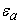,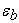,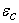(Figure 4).

Table 1 lists strains which were measured around the hole in individual drilling steps in position 5. The output strain values help us determine principal normal stresses in the point of drilling.Download asVeiw figureFigures index
Figure 3. Hole drilling in position 5 on the transversal beamDownload asVeiw figureFigures index
Figure 4. Strain gauge in position 5

### 4. Application of the Polynomial Regression Models

The purpose of this analysis was to determine the relationship between strains,,in particular directions marked as a, b, c and hole depth h. The statistical analysis of the measured data was performed with using classical least squares theory and software MATLAB.

Figure 5 − Figure 7 presents a scatter diagrams of the strain values,,in particular directions marked as a, b, c versus drilling stage h with fitted regression polynomial models.Download asVeiw figureFigures index
Figure 5. Comparison of three polynomial regression models with measured data − direction aDownload asVeiw figureFigures index
Figure 6. Comparison of three polynomial regression models with measured data − direction bDownload asVeiw figureFigures index
Figure 7. Comparison of four polynomial regression models with measured data − direction c

The basic statistical outputs for particular directions a, b, c are, respectively, shown in Table 2Table 4

#### Table 4. Regression statistics for direction c

The root mean squared error RMSE is an estimator of the standard deviation σ of the random error term. RMSE is measure of the size of the errors in regression and do not give indication about the explained component of the regression fit.

The Rsquared R2 (coefficient of determination) measures percentage of variation in the dependent variable explained by the independent variable. The value of R2 is always between zero and one. An R2 value of 0.9 or above is very good, a value above 0.8 is good, and value of 0.6 or above may be satisfactory in some applications, although we must be aware of the fact, in such cases errors in prediction may be relatively high. When the R2 value is 0.5 or below, the regression explains only 50 % or less of the variation in the data; therefore, prediction may be poor. Adjusted Rsquared R*2 is adjusted for the number of variables included in the regression equation.

Mean absolute percentage error MAPE is the most useful measure to compare the accuracy of forecasts between different items or products since it measures relative performance. If MAPE calculated value is less than 10%, it is interpreted as excellent accurate forecasting, between 10 − 20 % good forecasting, between 20 − 50% acceptable forecasting and over 50% inaccurate forecasting .

The DurbinWatson (DW) statistic is used in a test for serial correlation of residuals (i.e., error terms) in simple or multiple regression models, and time series trend models. The DurbinWatson statistic is always between 0 and 4. The value DW of 2 means, that there is no autocorrelation in the sample. A value close to 0 indicates strong positive correlation, while a value of 4 indicates strong negative correlation. An acceptable range is 1.4 − 2.6 [3, 4].

There is a test that uses the tdistribution to test single parameters of the regression model. Let's set the null hypothesis H0: βj = 0, and the alternative hypothesis H1: βj ≠ 0,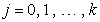.  Software MATLAB prints all these tests and the corresponding pvalues (see Table 5Table 7). We used significance level α = 0.05.

#### Table 7. Pvalues of ttests for direction c

Direction a: In Table 2 and Table 5 we can see that the quadratic regression polynomial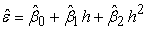fit the measured data very good. Symbols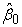,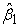, and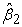are unbiased estimators of the true regression coefficients β0, β1, and β2. Least squares parameter estimates for this model: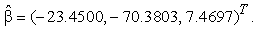Direction b: The quadratic polynomial regression model is here the best (see Table 3 and Table 6). Least squares parameter estimates for this model: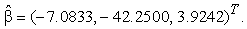Direction c: We find that the cubic polynomial regression model appears to fit the data best (see Table 4 and Table 7). Least squares parameter estimates for this model: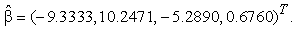Estimation of the model parameters requires the assumption that the errors are uncorrelated random variables normally distributed with mean zero and constant variance σ2. The random error terms are estimated by the residuals − the differences between the observed values and the fitted values. The analysis of residuals plays an important role in validating the regression model. Graphical analysis is much more effective in trying to detect patterns in the residuals than looking at the raw numbers. It may also be useful to plot standardized residuals vs. fitted values (Figure 8). The residuals would have to be randomly distributed around zero. If the errors are norm(0,σ 2), then approximately 95 % of the standardized residuals should fall in the interval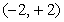.

The residuals for directions a, b, c in Figure 9 show no curvature or changing variance. The residual plots show a fairly random pattern that indicates models provide a good fit to the data.

An important application of a regression model is predicting new or future observations of strains,,corresponding to a specified level of the independent variable h. We can compute e.g. 95 % prediction interval for strains,,in particular directions marked as a, b, c. Figure 9 − Figure 11 show the 95 % prediction interval for strains in particular directions by using the best polynomial regression model.Download asVeiw figureFigures index
Figure 8. Scatter plots of standardized residuals vs. fitted values for directions a, b, cDownload asVeiw figureFigures index
Figure 9. 95% prediction interval using quadratic polynomial−direction aDownload asVeiw figureFigures index
Figure 10. 95% prediction interval using quadratic polynomial−direction bDownload asVeiw figureFigures index
Figure 11. 95 % prediction interval using cubic polynomial − direction c

### 5. Conclusion

Measured strains in positions 1 − 5 on the structure (Figure 2), principal normal stresses, which were determined on the basis of these strains, and their gradients demonstrate that principal normal stresses are tensile stresses. With respect to occurrence and spread of cracks, such stresses indicate the most critical case. On the analyzed surface these stresses reach the limit of yield strength and cause plastic deformations. These deformations combine with stresses and superpose to operational loading. Direction offset angles of bigger residual stresses from the reference axis of strain gauges confirm the initial point of crack spread. The occurrence of cracks in positions 1, 3 and 4 was caused by high stresses and a different curvature radius of the stress concentrator which in this case was the weld.

These results point out, that such high values of residual stresses have considerably affected the life of the bearing structure. Based on the abovementioned, structural changes have been made to decrease stresses which affect working life of the structure.

By means of mathematical statistics we were able to identify the tendency of strain changes,,relative to the depth of drilling. These changes can be seen in Figure 9 − Figure 11. The abovementioned statistical analysis and the application of polynomial regression models were used to verify the chosen procedure. Such procedure can be used for future verification of similar tasks.

### Acknowledgements

This work was supported by the grant projects VEGA No. 1/0872/16, VEGA 1/0731/16 and project APVV 15-0435.

### References

  Trebuňa, F., Šimčák, F., Handbook of Experimental Mechanics (in Slovak), TypoPress, Košice, 2007.In article  Makridakis, S., Wheelwright, S.C., Forecasting methods and applications, John Wiley & Sons, New York, 1998.In article  Montgomery, D.C., Runger, G.C., Applied Statistics and Probability for Engineers, John Wiley & Sons, 2003.In article  Ostertagová, E., Modelling Using Polynomial Regression, Procedia Engineering, 48 (2012), p. 500-506.In article View Article  Ostertagová, E., Applied Statistics in the Computational Environment of the MATLAB software (in Slovak), TU Košice, 2015.In article